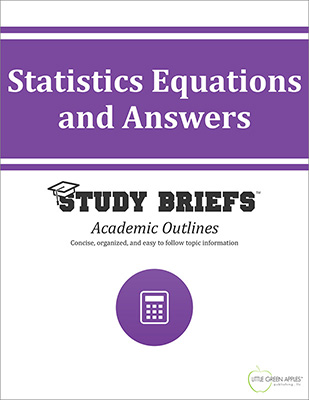Look inside
• Format: eBook
• Edition: 2016
• Publisher: Little Green Apples Publishing LLC

Price: \$4.99

## Description:

Need some help solving statistics problems? Here's an answer! A highly reliable resource created by our educational experts. This handy compilation of need-to-know Statistics formulas, equations and answers is a concise yet thorough reference guide. It is recommended as an advanced level companion to our Statistics Study Brief. This short guide complies a wealth of information into an everyday reference including the most up to date figures found iin current textbooks and online resources. It provides quick access to definitions of key terms and explanations of fundamental principles supported by instructive examples and clarifying visuals. Inside you will find numerous useful examples of formulas and equations related to mean, median, mode, standard deviation and variance, quartiles, normal distribution, frequency distribution, statistical reference sampling, linear regression, chi-square statistic, probability (including basic rules and models), Venn diagrams, disjoint and overlapping events, combination and permutation, confidence intervals, test of significance, analysis of variance, multivariate analysis of variance (MANOVA), and Type I and Type II errors. Having at your fingertips for quick and easy reference, the numerous real-world examples of statistical formulas, equations and answers makes this Study Brief an invaluable resource for your personal library.

## Topics Covered:

• What is Statistics?
• Finding the Mean in a Data Set
• Finding the Median in a Data Set
• Finding the Mode in a Data Set
• Standard Deviation and Variance
• Quartiles
• Normal Distribution
• Frequency Distribution
• Statistical Inference Sampling
• Linear Regression
• Chi-Square Statistic
• Probability
• Basic Rules of Probability
• Conditional and Independent Probability
• Binomial or Repeated Trial Probability
• Venn Diagram
• Disjoint and Overlapping Events
• Combination and Permutation
• Confidence Intervals
• Tests of Significance
• Analysis of Variance (ANOVA)
• Multivariate Analysis of Variance (MANOVA)
• Type I and Type II Errors

Browse Subjects